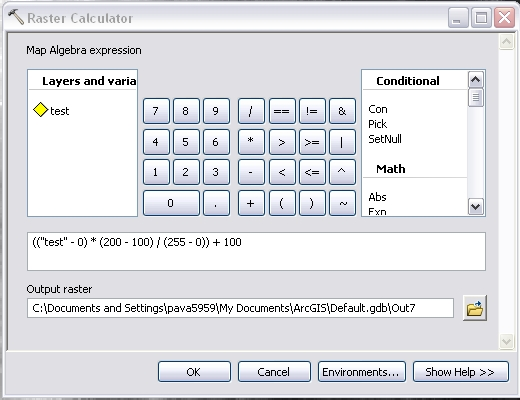English

# How To: Rescale raster data

## Summary

Instructions provided explain how to rescale values from one raster into a new raster so that the shape (distribution) of the raster's histogram (pixel values) does not change.

`Note:The Spatial Analyst extension is needed for ArcGIS to scale the data following the methods listed below.`

## Procedure

1. Open the Raster Calculator from the Spatial Analyst toolbar. The Single Output Map Algebra tool can also be used.
2. Determine the maximum and minimum values used to scale the data.
3. Use the following equation to scale the grid values:

`Rescaled grid = [(grid - Min value from grid) * (Max scale value - Min scale value) / (Max value from grid - Min value from grid)] + Min scale value`

Example 1:
Scale from 0-255 (8-bit range) to 0-65535 (16-bit range).
For ArcGIS 9.x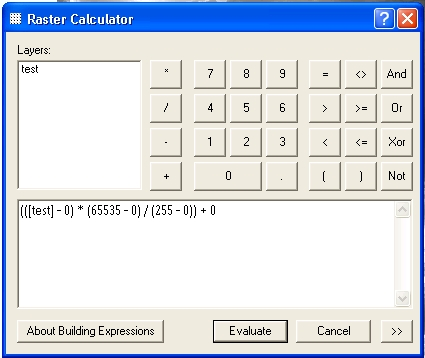For ArcGIS 10.x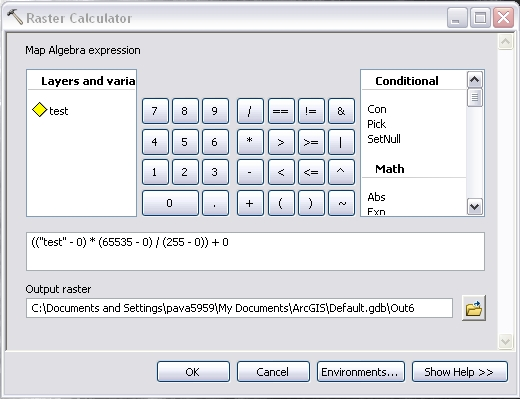Example 2:
Scale from 0-65535 (16-bit range) to 0-255 (8-bit range).
For ArcGIS 9.x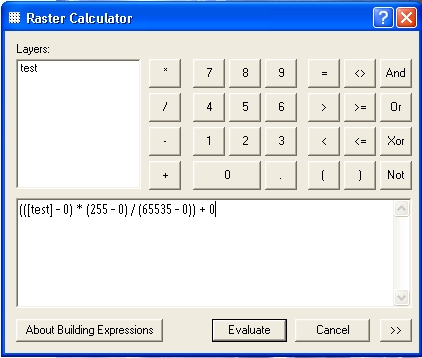For ArcGIS 10.x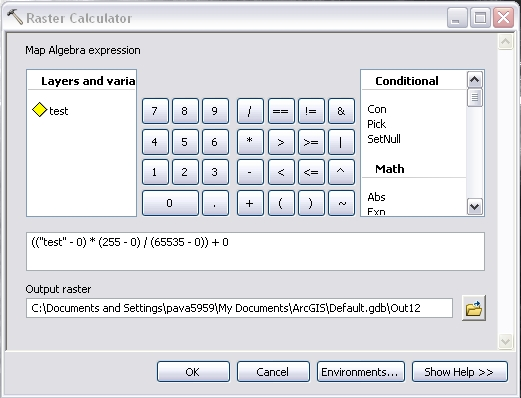Example 3:
Scale from range of 0-255 to range of 100-200.
For ArcGIS 9.x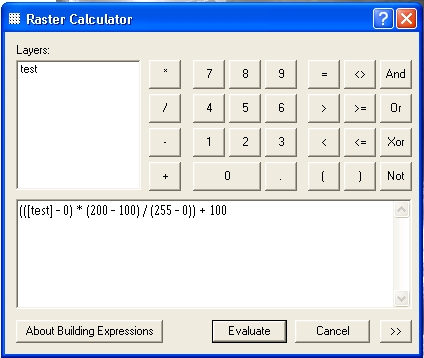For ArcGIS 10.x